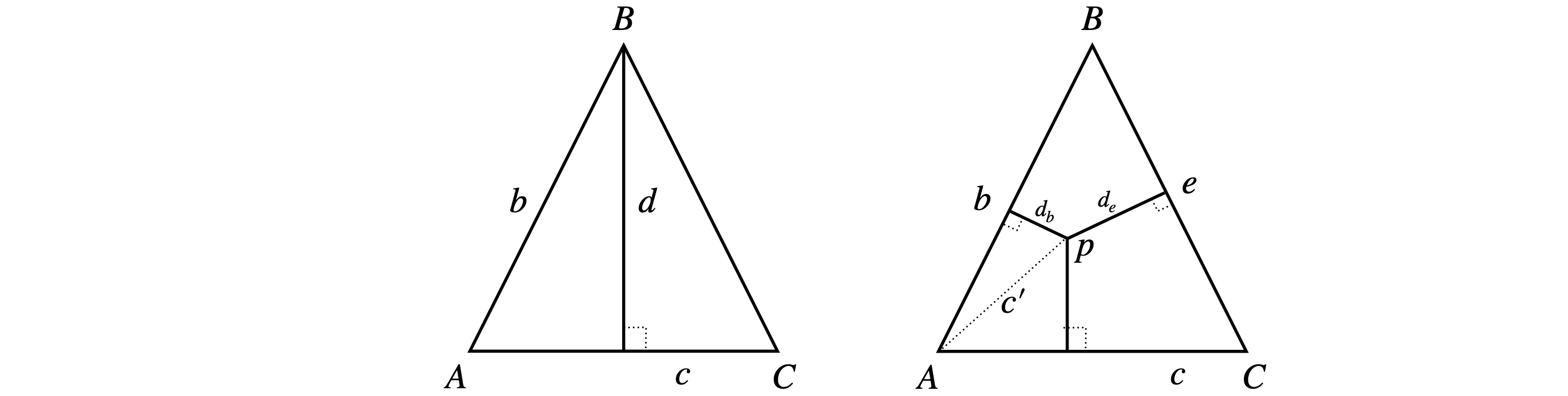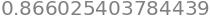# Solutions Q47 - 52#

# import all python add-ons etc that will be needed later on
%matplotlib inline
import numpy as np
import matplotlib.pyplot as plt
from sympy import *
init_printing()                      # allows printing of SymPy results in typeset maths format
plt.rcParams.update({'font.size': 16})  # set font size for plots


Start by defining vectors in the $$\boldsymbol i-\boldsymbol j$$ plane as

$\displaystyle \vec a = \cos(\alpha)\;\boldsymbol i + \sin(\alpha)\;\boldsymbol j,\quad \vec b = \cos(\beta)\;\boldsymbol i + \sin(\beta)\;\boldsymbol j$

Recall that as $$\boldsymbol i \times \;\boldsymbol i = \;\boldsymbol j \times \;\boldsymbol j = 0$$ and $$\boldsymbol j \times \;\boldsymbol i = \boldsymbol -k$$ the cross product is;

$\displaystyle \vec a \times \vec b = \big(\cos(\alpha)\;\boldsymbol i + \sin(\alpha)\;\boldsymbol j\big) \times \big(\cos(\beta)\;\boldsymbol i + \sin(\beta)\;\boldsymbol j\big) = \cos(\alpha)\sin(\beta)\;\boldsymbol k - \sin(\alpha)\cos(\beta)\;\boldsymbol k$

which, as it has components in $$\boldsymbol k$$ only, must be perpendicular to $$\vec a$$ and $$\vec b$$.

In matrix form, the calculation is clearer

$\begin{split}\displaystyle \begin{vmatrix}\boldsymbol i & \boldsymbol j & \boldsymbol k\\\cos(\alpha) & \sin(\alpha) &0\\\cos(\beta) & \sin(\beta) & 0 \end{vmatrix}= \cos(\alpha)\sin(\beta)\;\boldsymbol k - \sin(\alpha)\cos(\beta)\;\boldsymbol k\end{split}$

Secondly, as $$\vec a\times \vec b=|\vec a||\vec b|\sin(\alpha -\beta)\;\boldsymbol k$$ and as the magnitude $$|\vec a| = |\vec b| = 1$$ then $$\vec a\times \vec b = \sin(\alpha -\beta)\;\boldsymbol k$$. The vector $$\boldsymbol k$$ is present because vectors $$\vec a$$ and $$\vec b$$ are entirely in the $$i-j$$ plane and $$\vec a\times \vec b$$ is perpendicular to this plane. Comparing the two definitions of $$\vec a\times \vec b$$ proves the relationship.

By definition, $$\vec A\times\vec B =ab\sin(\chi)$$ and similarly for other sides, then

$\displaystyle ab\sin(\chi) = ca\sin(\beta) = bc\sin(\alpha)\quad\text{ or }\quad \displaystyle \frac{\sin(\alpha)}{a}=\frac{\sin(\beta)}{b}=\frac{\sin(\chi)}{c}$

which is the ‘law of sines’.Figure 76.

If you want to do the calculation by hand,the basis set to use is the orthogonal set $$(i, j, k)$$. The vectors can be defined as $$\vec A = a_1\;\boldsymbol i + a_2\;\boldsymbol j + a_3\;\boldsymbol k$$ and a similar equation for $$\vec B$$ and $$\vec C$$ with coefficients $$b_{1-3},\; c_{1-3}$$. Alternatively matrices can be used. In Python/Sympy we need to specify the vectors as one-dimensional matrices. The calculation is simple as dot and cross products are pre-defined.

a1, a2, a3, b1, b2, b3, c1, c2, c3 = symbols('a1, a2, a3, b1, b2, b3, c1, c2, c3', real=True)

A = Matrix( [a1, a2, a3] )
B = Matrix( [b1, b2, b3] )
C = Matrix( [c1, c2, c3] )

f1 = A.cross( B.cross(C) )      # A x( B x C)
f2 = B*(A.dot(C))               # B(A.C)
f3 = C*(A.dot(B))               # C(A.B)
simplify( f1 - f2 + f3 )

$\begin{split}\displaystyle \left[\begin{matrix}0\\0\\0\end{matrix}\right]\end{split}$

(a) In this calculation, the line is $$Ap$$, and the line $$Bp$$ is at an angle $$\theta$$ from $$Ap$$. Defining the vectors, as in the example in the text, the distance of $$B$$ from $$Ap$$ can be found as follows using Python to do this numerically, or by hand. The calculation is similar to that in the text.

A = np.array( [1, -2, 3] )
B = np.array( [4,  6, 0] )
p = np.array( [1,  2, 3] )
a = p - A               # is a vector length 3
b = p - B
ab = np.cross(a,b)
# print('ab =',ab)
d = np.sqrt( np.dot(ab,ab) )/np.sqrt( np.dot(a,a) )
print('{:s}{:6.3f}'.format('d =',d ) )
d_B_Ap = np.sqrt( np.dot(ab,ab) )/np.sqrt( np.dot(b,b) )
print('{:s}{:6.3f}'.format('dist B to Ap =',d_B_Ap) )

d = 4.243
dist B to Ap = 2.910


(a) Let $$r$$ be the length of each side. If point A is at the origin, then C is at $$(r,0,0)$$ and B at $$(r/2,r\sqrt{3}/2,0)$$ as $$\sin(60^\text{o})=\sqrt{3}/2$$. The distance from $$B$$ to $$AC$$ is $$\displaystyle |\vec d|=\frac{|\vec b \times \vec c|}{|\vec c|}$$Figure 77. Calculating the length of perpendiculars.

As the length $$|\vec c|=r$$ then

$\displaystyle \vec c=r\;\boldsymbol i+0\;\boldsymbol j+0\;\boldsymbol k,\quad \vec b=\frac{r}{2}\;\boldsymbol i+\frac{r\sqrt{3}}{2}\;\boldsymbol j+0\;\boldsymbol k$

and the cross product

$\begin{split}\displaystyle \vec b\times\vec c=\begin{vmatrix}\;\boldsymbol i&\;\boldsymbol j&\;\boldsymbol k\\r/2& r\sqrt{3}/2 & 0\\r&0&0\end{vmatrix}=0\;\boldsymbol i-0\;\boldsymbol j-\frac{r^2\sqrt{3}}{2}\;\boldsymbol k\end{split}$

and the distance from $$B \to AC$$ is therefore $$d=r\sqrt{3}/2$$ and by symmetry this is also the length of the other perpendiculars. This could,of course, have been calculated more easily using simple geometry.

(b) To calculate the length $$d_b$$,find vector $$\vec c'$$ (right hand side of figure 77) and calculated $$\displaystyle d_b=\frac{|\vec b \times \vec c'|}{|\vec b|}$$. Let the vector $$\vec c'=\begin{bmatrix}x&y&0\end{bmatrix}$$ and because $$A$$ is at the origin and $$p$$ a point with coordinates $$(x,y,0)$$ and thus from part (a)

$\begin{split}\displaystyle \vec b\times\vec{c'}=\begin{vmatrix}\;\boldsymbol i&\;\boldsymbol j&\;\boldsymbol k\\r/2& r\sqrt{3}/2 & 0\\x&y&0\end{vmatrix}=0\;\boldsymbol i-0\;\boldsymbol j+(y-x)\frac{r\sqrt{3}}{2})\;\boldsymbol k\end{split}$

making length $$\displaystyle d_b=\left|(y-x)\frac{\sqrt{3}}{2}\right|$$ and the absolute value is used as the length must be positive.

The length $$p\to \vec c$$ (line $$AC$$) is $$d_c=y$$ by a similar calculation using $$(\vec c\times \vec{c'})$$. This also is clear from the diagram since $$AC$$ lies along the $$x$$-axis. The length $$d_e$$ is found by calculating the vector $$\vec{pC}$$ and vector $$\vec e$$. The result is

$\begin{split}\displaystyle \vec {pC}\times\vec{c'}=\begin{vmatrix}\;\boldsymbol i&\;\boldsymbol j&\;\boldsymbol k\\x-r& y & 0\\-r/2&r\sqrt{3}/2&0\end{vmatrix}=0\;\boldsymbol i-0\;\boldsymbol j+\frac{r}{2}\left(\sqrt{3}(x-r)+y\right)\;\boldsymbol k\end{split}$

giving a length $$\displaystyle d_e = \left|\frac{\sqrt{3}(x-r)}{2} +\frac{y}{2} \right|$$. The total distance of all perpendiculars is the sum of the three terms,$$d_c+b_B+d_e$$.

To test this with some values Python can be used with the restriction that $$(x,y)$$ must lie within the triangle, that is when $$x\le r/2,\;y\le x\sqrt{3}/2$$ and when $$\displaystyle x\ge \sqrt{3}/2,\; y\le -\frac{\sqrt{3}}{2}(x-r/s)+r\sqrt{3}/4$$

# check on distaces
r = 1
f = lambda x,y : np.abs(y/2-x*np.sqrt(3)/2) + y + np.abs((x - r)*np.sqrt(3)/2 + y/2)

f(1/4,1/8)and the same answer $$0.866=\sqrt(3)/2$$ is obtained for other valid $$x,\;y$$ which confirms the value given in the question.

Using Python to do the arithmetic, calculate the bond distances first. It is not obvious from the data which O atom to take, so calculate both distances. A simple function ‘length’ is made to calculate the lengths to avoid retyping similar equations.

length = lambda v: np.sqrt(np.dot(v, v))

Fe   = np.array([6.591, -16.663,  12.091])        #pdb 1THB. HETATM 1THN3507, HEM C 1
HisN = np.array([7.742, -15.655,  10.429])        # HIS 87 NE2 1THB3086
NA   = np.array([7.559, -18.441,  12.096])
NB   = np.array([7.832, -16.106,  13.651])
NC   = np.array([5.405, -15.044,  12.620])
ND   = np.array([5.015, -17.506,  11.076])
Ox1  = np.array([5.558, -17.474,  13.668])
Ox2  = np.array([4.756, -17.191,  14.691])

FeOx1 = Fe - Ox1
FeOx2 = Fe - Ox2
FeHisN= Fe-HisN

print('{:s}{:6.3f}'.format('bond length Fe-Ox1', length(FeOx1)) )
print('{:s}{:6.3f}'.format('bond length Fe-Ox2', length(FeOx2)) )
print('{:s}{:6.3f}'.format('bond length Fe-HisN',length(FeHisN)) )

# calc eqn of plane

NANB = NA - NB
NAND = NA - ND
n    = np.cross(NANB,NAND)            # n is normal to plane of N atoms A, B, C
X0   = NA                             # is [NA[o],NA,NA] but any point in plane can be used
dn   = length(n)
d    = np.dot(n,Ox1-X0)/dn            # distance Ox1 to plane of N atoms
print('{:s}{:8.3f}'.format('distance Ox1 to plane', d ) )
d    = np.dot(n,Ox2-X0)/dn
print('{:s}{:8.3f}'.format('distance Ox2 to plane', d ) )
d    = np.dot(n,HisN-X0)/dn
print('{:s}{:8.3f}'.format('distance HisN to plane', d ) )
d    = np.dot(n,Fe-X0)/dn
print('{:s}{:8.3f}'.format('distance Fe to plane', d ) )

bond length Fe-Ox1 2.052
bond length Fe-Ox2 3.226
bond length Fe-HisN 2.259
distance Ox1 to plane   1.698
distance Ox2 to plane   2.724
distance HisN to plane  -2.607
distance Fe to plane  -0.350

# get equation of plane with Sympy
x, y, z = symbols('x, y, z')  # use sympy as xyz are symbols
X = Matrix( [x, y, z] )
X0= Matrix( [NA, NA, NA] )  # any point in plane
plane= np.dot(n,X - X0)              # n is defined above
print(plane)

[-3.83562499999999*x - 3.67746*y + 6.19549499999999*z - 113.763258005]


From these results, the oxygen atom Ox1 is clearly attached to the Fe atom, which is $$0.35$$ angstrom out of the plane of the three porphyrin atoms. Because the Fe-Ox1 distance is larger than the plane to OX1 distance, the Fe atom is out of the plane on the side towards the Histidine group, not the oxygen; this is clear from the sign of the distances also.

Fe-O distances in inorganic molecules are in the range $$1.9 \to 2.1$$ and Fe-N $$1.95 \to 1.99$$ Angstrom.

Exercise: Find an X-ray structure of deoxygenated haemoglobin in the PDB, and compare the Fe-O and FeN(His) distances with those calculated in this problem.